• 自己动手证明向量点乘和叉乘的几何意义 数学

对于游戏行业程序员来说，向量点乘”和“叉乘”是非常熟悉的运算。从代码上看他们很简单：（以下代码选自UE4的“Vector.h”） 点乘就是各分量逐项相乘，最终得到了一个标量： FORCEINLINE float FVector::Dot...

目标

对于游戏行业程序员来说，向量“点乘”和“叉乘”是非常熟悉的运算。从代码上看他们运算过程并不复杂：（以下代码选自UE4的“Vector.h”）

点乘就是各分量逐项相乘，最终得到了一个标量

FORCEINLINE float FVector::DotProduct(const FVector& A, const FVector& B)
{
return X*V.X + Y*V.Y + Z*V.Z;
}

叉乘最终得到一个新的向量，虽然其运算现在看起来略显“奇怪”（不过，在随后的证明中可以看出其重要的几何意义）：

FORCEINLINE FVector FVector::CrossProduct(const FVector& A, const FVector& B)
{
return FVector
(
Y * V.Z - Z * V.Y,
Z * V.X - X * V.Z,
X * V.Y - Y * V.X
);
}

即：
a ⃗ ⋅ b ⃗ = x a x b + y a y b + z a z b \vec{a}\cdot\vec{b} = x_ax_b+y_ay_b+z_az_b

a ⃗ × b ⃗ = ( y a z b − z a y b , z a x b − x a z b , x a y b − y a x b ) \vec{a}\times\vec{b} = (y_az_b-z_ay_b,z_ax_b-x_az_b,x_ay_b-y_ax_b)

他们各自都有重要的几何意义，经常会出现在有关空间计算的逻辑中，例如：

• 标准化后向量点乘得到的值为夹角的余弦。这样，只要计算点乘，得到-1~ 0 ~1，便可知道两个向量之间方向的关系是相反 ~ 垂直 ~ 相同
• 向量叉乘后得到的新向量一定和原先两个向量垂直。
• 向量叉乘后得到的向量的模，其值为两个向量构成的三角形的面积的二倍。

向量点乘和叉乘的这些特性经常被使用，但是对于其中的数学原理我是模糊不清的，因此我想自己动手证明一下。

而为了让这些证明得更容易，我还需要在此之前证明其基于的一些定理。

1. 证明：勾股定理

定理：
对于一个直角三角形ABC，其中直角为∠BAC，有：
B C 2 = A B 2 + A C 2 BC^2=AB^2+AC^2

其证明网上有很多种，下面的方法据说来自于欧几里得的《几何原本》。（图片来源勾股定理_百度百科

由于 三角形FBC正方形GFBA 同底等高，所以有：
S F B C × 2 = S G F B A S_{FBC}\times2=S_{GFBA}

由于 三角形FBC三角形ABD 为全等三角形，所以有：
S F B C = S A B D S_{FBC}=S_{ABD}

由于 三角形ABD矩形BDLK 同底等高，所以有：
S A B D × 2 = S B D L K S_{ABD}\times2=S_{BDLK}

因此：
S G F B A = S F B C × 2 = S A B D × 2 = S B D L K S_{GFBA}=S_{FBC}\times2=S_{ABD}\times2=S_{BDLK}

同理：
S A C I H = S K L E C S_{ACIH}=S_{KLEC}

（即图中粉色正方形的面积等于粉色矩形的面积，蓝色正方形的面积等于蓝色矩形的面积）

因此：
B C 2 = S B D E C = S B D L K + S K L E C = S G F B A + S A C I H = A B 2 + A C 2 \begin{aligned} BC^2 & =S_{BDEC}\\ & =S_{BDLK}+S_{KLEC}\\ & =S_{GFBA}+S_{ACIH}\\ & = AB^2+AC^2\\ \end{aligned}

2. 证明：余弦定理

定理：
对于任意一个三角形ABC，有：
A B 2 = B C 2 + A C 2 − 2 B C ⋅ A C ⋅ c o s C AB^2=BC^2+AC^2-2BC \cdot AC \cdot cosC

证明网上也有很多种，下面的方法据说也来自于欧几里得的《几何原本》。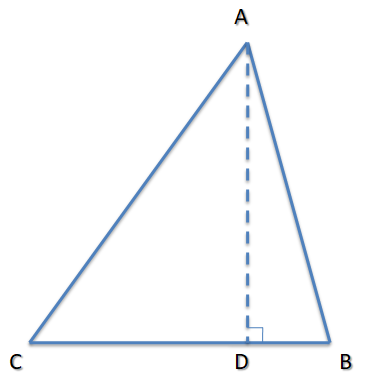A D = A C ⋅ s i n C AD = AC \cdot sinC

C D = A C ⋅ c o s C CD = AC \cdot cosC

在CB边上，有：
D B = B C − C D = B C − A C ⋅ c o s C DB= BC-CD=BC- AC \cdot cosC

A B 2 = A D 2 + D B 2 = ( A C ⋅ s i n C ) 2 + ( B C − A C ⋅ c o s C ) 2 = A C 2 ⋅ s i n C 2 + B C 2 + A C 2 ⋅ c o s C 2 − 2 B C ⋅ A C ⋅ c o s C = B C 2 + A C 2 ⋅ s i n C 2 + A C 2 ⋅ c o s C 2 − 2 B C ⋅ A C ⋅ c o s C = B C 2 + A C 2 ( s i n C 2 + c o s C 2 ) − 2 B C ⋅ A C ⋅ c o s C = B C 2 + A C 2 − 2 B C ⋅ A C ⋅ c o s C \begin{aligned} AB^2 & =AD^2+DB^2\\ & =(AC \cdot sinC)^2+(BC- AC \cdot cosC)^2\\ & =AC^2\cdot sinC^2+BC^2+AC^2\cdot cosC^2-2BC \cdot AC \cdot cosC\\ & =BC^2+AC^2\cdot sinC^2+AC^2\cdot cosC^2-2BC \cdot AC \cdot cosC\\ & =BC^2+AC^2(sinC^2+cosC^2)-2BC \cdot AC \cdot cosC\\ & =BC^2+AC^2-2BC \cdot AC \cdot cosC\\ \end{aligned}

3. 证明：向量点乘的几何意义——结果为模相乘再乘夹角余弦

向量点乘的定义如下：
a ⃗ ⋅ b ⃗ = x a x b + y a y b + z a z b \vec{a}\cdot\vec{b} = x_ax_b+y_ay_b+z_az_b

现在想证明的是：（其中θ为两向量夹角）
a ⃗ ⋅ b ⃗ = ∥ a ⃗ ∥ ⋅ ∥ b ⃗ ∥ c o s θ \vec{a}\cdot\vec{b} =\left \| \vec{a} \right \|\cdot\left \| \vec{b} \right \|cos\theta

在证明前，先看一个算不上是“定理”的结论：
对于一个2维的向量(x,y)，由于坐标系是垂直的，所以由勾股定理很容易能推导出
2 维 向 量 长 度 2 = x 2 + y 2 2维向量长度^2=x^2+y^2

对于一个3维的向量(x,y,z)，也可以很容易能推导出：
3 维 向 量 长 度 2 = x 2 + y 2 + z 2 3维向量长度^2=x^2+y^2+z^2

下面正式开始证明：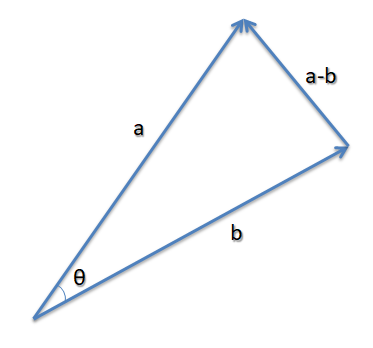如果将向量a和向量b的起点放在一起，那么这两个向量终点之间的向量即为 a-b

而根据余弦定理，有：
∥ a ⃗ − b ⃗ ∥ 2 = ∥ a ⃗ ∥ 2 + ∥ b ⃗ ∥ 2 − 2 ∥ a ⃗ ∥ ⋅ ∥ b ⃗ ∥ c o s θ \left \| \vec{a} -\vec{b} \right \|^2=\left \| \vec{a} \right \|^2 +\left \| \vec{b} \right \|^2 -2\left \| \vec{a} \right \|\cdot\left \| \vec{b} \right \|cos\theta
写成分量的形式，就是：
( ( x a , y a , z a ) − ( x b , y b , z b ) ) 2 = ( x a , y a , z a ) 2 + ( x b , y b , z b ) 2 − 2 ∥ a ⃗ ∥ ⋅ ∥ b ⃗ ∥ c o s θ ((x_a,y_a,z_a)-(x_b,y_b,z_b))^2=(x_a,y_a,z_a)^2+(x_b,y_b,z_b)^2-2\left \| \vec{a} \right \|\cdot\left \| \vec{b} \right \|cos\theta

向量相减即各分量相减，即：
( x a − x b , y a − y b , z a − z b ) 2 = ( x a , y a , z a ) 2 + ( x b , y b , z b ) 2 − 2 ∥ a ⃗ ∥ ⋅ ∥ b ⃗ ∥ c o s θ (x_a-x_b,y_a-y_b,z_a-z_b)^2=(x_a,y_a,z_a)^2+(x_b,y_b,z_b)^2-2\left \| \vec{a} \right \|\cdot\left \| \vec{b} \right \|cos\theta

将平方的运算展开：
x a 2 + x b 2 − 2 x a x b + y a 2 + y b 2 − 2 y a y b + z a 2 + z b 2 − 2 z a z b = x a 2 + x b 2 + y a 2 + y b 2 + z a 2 + z b 2 − 2 ∥ a ⃗ ∥ ⋅ ∥ b ⃗ ∥ c o s θ x_a^2+x_b^2-2x_ax_b+y_a^2+y_b^2-2y_ay_b+z_a^2+z_b^2-2z_az_b=x_a^2+x_b^2+y_a^2+y_b^2+z_a^2+z_b^2-2\left \| \vec{a} \right \|\cdot\left \| \vec{b} \right \|cos\theta

去掉等式两边重复项可得：
− 2 x a x b − 2 y a y b − 2 z a z b = − 2 ∥ a ⃗ ∥ ⋅ ∥ b ⃗ ∥ c o s θ -2x_ax_b-2y_ay_b-2z_az_b=-2\left \| \vec{a} \right \|\cdot\left \| \vec{b} \right \|cos\theta

约去-2得：
x a x b + y a y b + z a z b = ∥ a ⃗ ∥ ⋅ ∥ b ⃗ ∥ c o s θ x_ax_b+y_ay_b+z_az_b=\left \| \vec{a} \right \|\cdot\left \| \vec{b} \right \|cos\theta

结合向量点乘的定义，则最后可知：
a ⃗ ⋅ b ⃗ = x a x b + y a y b + z a z b = ∥ a ⃗ ∥ ⋅ ∥ b ⃗ ∥ c o s θ \begin{aligned} \vec{a}\cdot\vec{b} & =x_ax_b+y_ay_b+z_az_b \\ & =\left \| \vec{a} \right \|\cdot\left \| \vec{b} \right \|cos\theta \\ \end{aligned}

4. 证明：向量叉乘的几何意义——结果与原先两个向量都垂直

向量叉乘的定义如下：
a ⃗ × b ⃗ = ( y a z b − z a y b , z a x b − x a z b , x a y b − y a x b ) \vec{a}\times\vec{b} = (y_az_b-z_ay_b,z_ax_b-x_az_b,x_ay_b-y_ax_b)

由前面证明的向量点乘的几何意义可知，
如果能证明：

( a ⃗ × b ⃗ ) ⋅ a ⃗ = 0 (\vec{a}\times\vec{b} )\cdot \vec{a}=0

( a ⃗ × b ⃗ ) ⋅ b ⃗ = 0 (\vec{a}\times\vec{b} )\cdot \vec{b}=0

则意味着向量叉乘的结果向量，和原先两个向量的夹角的余弦值都为0，即夹角为90°，即与原先两个向量都垂直

而这个证明可以直接从算式中得出：
( a ⃗ × b ⃗ ) ⋅ a ⃗ = ( y a z b − z a y b , z a x b − x a z b , x a y b − y a x b ) ⋅ ( x a , y a , z a ) = y a z b x a − z a y b x a + z a x b y a − x a z b y a + x a y b z a − y a x b z a = ( y a z b x a − x a z b y a ) + ( x a y b z a − z a y b x a ) + ( z a x b y a − y a x b z a ) = 0 + 0 + 0 = 0 \begin{aligned} (\vec{a}\times\vec{b} )\cdot \vec{a} & = (y_az_b-z_ay_b,z_ax_b-x_az_b,x_ay_b-y_ax_b) \cdot (x_a,y_a,z_a) \\ & =y_az_bx_a-z_ay_bx_a+z_ax_by_a-x_az_by_a+x_ay_bz_a-y_ax_bz_a\\ & =(y_az_bx_a-x_az_by_a)+(x_ay_bz_a-z_ay_bx_a)+(z_ax_by_a-y_ax_bz_a)\\ & =0+0+0\\ & =0\\ \end{aligned}

( a ⃗ × b ⃗ ) ⋅ b ⃗ = ( y a z b − z a y b , z a x b − x a z b , x a y b − y a x b ) ⋅ ( x b , y b , z b ) = y a z b x b − z a y b x b + z a x b y b − x a z b y b + x a y b z b − y a x b z b = ( y a z b x b − y a x b z b ) + ( z a x b y b − z a y b x b ) + ( x a y b z b − x a z b y b ) = 0 + 0 + 0 = 0 \begin{aligned} (\vec{a}\times\vec{b} )\cdot \vec{b} & = (y_az_b-z_ay_b,z_ax_b-x_az_b,x_ay_b-y_ax_b) \cdot (x_b,y_b,z_b) \\ & =y_az_bx_b-z_ay_bx_b+z_ax_by_b-x_az_by_b+x_ay_bz_b-y_ax_bz_b\\ & =(y_az_bx_b-y_ax_bz_b)+(z_ax_by_b-z_ay_bx_b)+(x_ay_bz_b-x_az_by_b)\\ & =0+0+0\\ & =0\\ \end{aligned}

5. 证明：向量叉乘的几何意义——结果的模为原先两向量的模相乘再乘夹角正弦

向量叉乘的定义如下：
a ⃗ × b ⃗ = ( y a z b − z a y b , z a x b − x a z b , x a y b − y a x b ) \vec{a}\times\vec{b} = (y_az_b-z_ay_b,z_ax_b-x_az_b,x_ay_b-y_ax_b)

现在想证明的是：（其中θ为两向量夹角）
∥ a ⃗ × b ⃗ ∥ = ∥ a ⃗ ∥ ∥ b ⃗ ∥ s i n θ \left \|\vec{a}\times\vec{b}\right \| =\left \| \vec{a} \right \|\left \| \vec{b} \right \|sin\theta

（方法来自于《3D游戏与计算机图形学中的数学方法》）

取向量叉乘结果的平方，逐步展开：
∥ a ⃗ × b ⃗ ∥ 2 = ( y a z b − z a y b , z a x b − x a z b , x a y b − y a x b ) 2 = ( y a z b − z a y b ) 2 + ( z a x b − x a z b ) 2 + ( x a y b − y a x b ) 2 = ( y a 2 z b 2 + z a 2 y b 2 − 2 y a z b z a y b ) + ( z a 2 x b 2 + x a 2 z b 2 − 2 z a x b x a z b ) + ( x a 2 y b 2 + y a 2 x b 2 − 2 x a y b y a x b ) = ( y a 2 z b 2 + z a 2 y b 2 + z a 2 x b 2 + x a 2 z b 2 + x a 2 y b 2 + y a 2 x b 2 ) + ( − 2 y a z b z a y b − 2 z a x b x a z b − 2 x a y b y a x b ) \begin{aligned} \left \|\vec{a}\times\vec{b}\right \| ^2 & = (y_az_b-z_ay_b,z_ax_b-x_az_b,x_ay_b-y_ax_b)^2 \\ & = (y_az_b-z_ay_b)^2+(z_ax_b-x_az_b)^2+(x_ay_b-y_ax_b)^2 \\ & = (y_a^2z_b^2+z_a^2y_b^2-2y_az_bz_ay_b)+(z_a^2x_b^2+x_a^2z_b^2-2z_ax_bx_az_b)+(x_a^2y_b^2+y_a^2x_b^2-2x_ay_by_ax_b) \\ & = (y_a^2z_b^2+z_a^2y_b^2+z_a^2x_b^2+x_a^2z_b^2+x_a^2y_b^2+y_a^2x_b^2)+(-2y_az_bz_ay_b-2z_ax_bx_az_b-2x_ay_by_ax_b)\\ \end{aligned}

下面便出现了我觉得这种证明方法比较“魔法”的一个操作，对于等号右侧：
左部分先加上了 ( x a 2 x b 2 + y a 2 y b 2 + z a 2 z b 2 ) (x_a^2x_b^2+y_a^2y_b^2+z_a^2z_b^2) ，右部分再减去它。一番“折腾”之后，虽然结果未受影响，但是却让左部分凑出了原先两向量的模的形式，右部分凑出了向量点乘的形式，具体来看：
∥ a ⃗ × b ⃗ ∥ 2 = ( y a 2 z b 2 + z a 2 y b 2 + z a 2 x b 2 + x a 2 z b 2 + x a 2 y b 2 + y a 2 x b 2 ) + ( x a 2 x b 2 + y a 2 y b 2 + z a 2 z b 2 ) + ( − 2 y a z b z a y b − 2 z a x b x a z b − 2 x a y b y a x b ) − ( x a 2 x b 2 + y a 2 y b 2 + z a 2 z b 2 ) = ( y a 2 z b 2 + z a 2 y b 2 + z a 2 x b 2 + x a 2 z b 2 + x a 2 y b 2 + y a 2 x b 2 + x a 2 x b 2 + y a 2 y b 2 + z a 2 z b 2 ) − ( x a 2 x b 2 + y a 2 y b 2 + z a 2 z b 2 + 2 y a z b z a y b + 2 z a x b x a z b + 2 x a y b y a x b ) = ( x a 2 + y a 2 + z a 2 ) ( x b 2 + y b 2 + z b 2 ) − ( x a x b + y a y b + z a z b ) 2 = ∥ a ⃗ ∥ 2 ∥ b ⃗ ∥ 2 − ( a ⃗ ⋅ b ⃗ ) 2 \begin{aligned} \left \|\vec{a}\times\vec{b}\right \| ^2 & = (y_a^2z_b^2+z_a^2y_b^2+z_a^2x_b^2+x_a^2z_b^2+x_a^2y_b^2+y_a^2x_b^2)+(x_a^2x_b^2+y_a^2y_b^2+z_a^2z_b^2)+(-2y_az_bz_ay_b-2z_ax_bx_az_b-2x_ay_by_ax_b)-(x_a^2x_b^2+y_a^2y_b^2+z_a^2z_b^2)\\ & = (y_a^2z_b^2+z_a^2y_b^2+z_a^2x_b^2+x_a^2z_b^2+x_a^2y_b^2+y_a^2x_b^2+x_a^2x_b^2+y_a^2y_b^2+z_a^2z_b^2)-(x_a^2x_b^2+y_a^2y_b^2+z_a^2z_b^2+2y_az_bz_ay_b+2z_ax_bx_az_b+2x_ay_by_ax_b)\\ & = (x_a^2+y_a^2+z_a^2)(x_b^2+y_b^2+z_b^2)-(x_ax_b+y_ay_b+z_az_b)^2\\ & =\left \| \vec{a} \right \|^2\left \| \vec{b} \right \|^2-(\vec{a}\cdot\vec{b})^2\\ \end{aligned}

而根据前面证明的向量点乘的几何意义可知：
a ⃗ ⋅ b ⃗ = ∥ a ⃗ ∥ ⋅ ∥ b ⃗ ∥ c o s θ \vec{a}\cdot\vec{b} =\left \| \vec{a} \right \|\cdot\left \| \vec{b} \right \|cos\theta

所以：
∥ a ⃗ × b ⃗ ∥ 2 = ∥ a ⃗ ∥ 2 ∥ b ⃗ ∥ 2 − ∥ a ⃗ ∥ 2 ∥ b ⃗ ∥ 2 ⋅ ( c o s θ ) 2 = ∥ a ⃗ ∥ 2 ∥ b ⃗ ∥ 2 ⋅ ( 1 − ( c o s θ ) 2 ) \begin{aligned} \left \|\vec{a}\times\vec{b}\right \| ^2 & = \left \| \vec{a} \right \|^2\left \| \vec{b} \right \|^2-\left \| \vec{a} \right \|^2\left \| \vec{b} \right \|^2\cdot (cos\theta)^2\\ & = \left \| \vec{a} \right \|^2\left \| \vec{b} \right \|^2\cdot (1- (cos\theta)^2) \end{aligned}

又因为【 ( c o s θ ) 2 + ( s i n θ ) 2 = 1 (cos\theta)^2+(sin\theta)^2=1 】一定成立
所以：
∥ a ⃗ × b ⃗ ∥ 2 = ∥ a ⃗ ∥ 2 ∥ b ⃗ ∥ 2 ⋅ ( s i n θ ) 2 \left \|\vec{a}\times\vec{b}\right \| ^2= \left \| \vec{a} \right \|^2\left \| \vec{b} \right \|^2\cdot (sin\theta)^2

开方得：
∥ a ⃗ × b ⃗ ∥ = ∥ a ⃗ ∥ ∥ b ⃗ ∥ ⋅ s i n θ \left \|\vec{a}\times\vec{b}\right \|= \left \| \vec{a} \right \|\left \| \vec{b} \right \|\cdot sin\theta

6. 证明：向量叉乘的几何意义——结果的模为原先两向量构成三角形的面积二倍

在前者证明之后，此证明变得很容易，因为：

∥ b ⃗ ∥ ⋅ s i n θ \left \| \vec{b} \right \|\cdot sin\theta 的长度就是以a为底边的三角形的高度：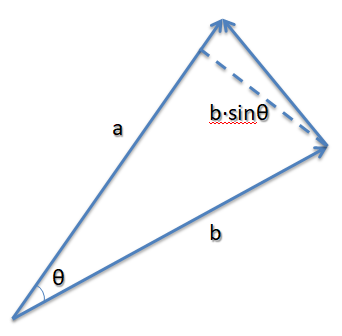所以：
∥ a ⃗ × b ⃗ ∥ = ∥ a ⃗ ∥ ∥ b ⃗ ∥ ⋅ s i n θ = ( ∥ a ⃗ ∥ ) × ( ∥ b ⃗ ∥ ⋅ s i n θ ) = 底 × 高 = 2 × ( 底 × 高 2 ) = 2 × S 三 角 形 \begin{aligned} \left \|\vec{a}\times\vec{b}\right \| & = \left \| \vec{a} \right \|\left \| \vec{b} \right \|\cdot sin\theta\\ & = (\left \| \vec{a} \right \|)\times (\left \| \vec{b} \right \|\cdot sin\theta)\\ & = 底 \times 高\\ & = 2\times (\frac{底 \times 高}{2})\\ & = 2\times S_{三角形} \end{aligned}

总结

向量点乘

定义

a ⃗ ⋅ b ⃗ = x a x b + y a y b + z a z b \vec{a}\cdot\vec{b} = x_ax_b+y_ay_b+z_az_b

几何意义与作用举例

a ⃗ ⋅ b ⃗ = ∥ a ⃗ ∥ ⋅ ∥ b ⃗ ∥ c o s θ \vec{a}\cdot\vec{b} =\left \| \vec{a} \right \|\cdot\left \| \vec{b} \right \|cos\theta

• 可以算出向量的夹角
• 可以直接根据此值判断两向量方向的关系：-1~ 0 ~1对应于相反 ~ 垂直 ~ 相同

向量叉乘

定义

a ⃗ × b ⃗ = ( y a z b − z a y b , z a x b − x a z b , x a y b − y a x b ) \vec{a}\times\vec{b} = (y_az_b-z_ay_b,z_ax_b-x_az_b,x_ay_b-y_ax_b)

几何意义与作用举例1

结果的向量与原先两个向量都垂直

• 可以用来快速算出两个向量确定的一个平面的法向量方向。
几何意义与作用举例2

∥ a ⃗ × b ⃗ ∥ = ∥ a ⃗ ∥ ∥ b ⃗ ∥ s i n θ \left \|\vec{a}\times\vec{b}\right \| =\left \| \vec{a} \right \|\left \| \vec{b} \right \|sin\theta

• 可以用来在已知顶点位置情况下，快速算出空间内一个三角形的面积。
展开全文u013412391 2021-01-22 21:43:03
• 向量点乘与叉乘 向量（Vector）  在几乎所有的几何问题中，向量（有时也称矢量）是一个基本点。向量的定义包含方向和一个数（长度）。在二维空间中，一个向量可以用一对x和y来表示。例如由点（1,3）到（5,1的向量...

向量点乘与叉乘

向量（Vector）
在几乎所有的几何问题中，向量（有时也称矢量）是一个基本点。向量的定义包含方向和一个数（长度）。在二维空间中，一个向量可以用一对x和y来表示。例如由点（1,3）到（5,1的向量可以用(4,-2）来表示。这里大家要特别注意，我这样说并不代表向量定义了起点和终点。向量仅仅定义方向和长度。

向量加法
向量也支持各种数学运算。最简单的就是加法。我们可以对两个向量相加，得到的仍然是一个向量。我们有：
V1（x1, y1）+V2（x2, y2）=V3(x1+x2, y1+y2)
下图表示了四个向量相加。注意就像普通的加法一样,相加的次序对结果没有影响（满足交换律），减法也是一样的。

点乘（Dot Product）
如果说加法是凭直觉就可以知道的，另外还有一些运算就不是那么明显的，比如点乘和叉乘。
点乘比较简单，是相应元素的乘积的和：
V1( x1, y1)   V2(x2, y2) = x1*x2 + y1*y2
注意结果不是一个向量，而是一个标量（Scalar）。点乘有什么用呢，我们有：
A   B = |A||B|Cos(θ)
θ是向量A和向量B见的夹角。这里|A|我们称为向量A的模(norm)，也就是A的长度， 在二维空间中就是|A| = sqrt(x2+y2)。这样我们就和容易计算两条线的夹角：    Cos(θ) = AB /(|A||B|)

当然你知道要用一下反余弦函数acos()啦。（回忆一下cos(90)=0 和cos(0) = 1还是有好处的，希望你没有忘记。）这可以告诉我们如果点乘的结果，简称点积，为0的话就表示这两个向量垂直。当两向量平行时，点积有最大值
另外，点乘运算不仅限于2维空间，他可以推广到任意维空间。（译注：不少人对量子力学中的高维空间无法理解，其实如果你不要试图在视觉上想象高维空间，而仅仅把它看成三维空间在数学上的推广，那么就好理解了）

叉乘（cross product）
相对于点乘，叉乘可能更有用吧。2维空间中的叉乘是：
V1(x1, y1) X V2(x2, y2) = x1y2 – y1x2
看起来像个标量，事实上叉乘的结果是个向量，方向在z轴上。上述结果是它的模。在二维空间里，让我们暂时忽略它的方向，将结果看成一个向量，那么这个结果类似于上述的点积，我们有：
A x B = |A||B|Sin(θ)

然而角度 θ和上面点乘的角度有一点点不同，他是有正负的，是指从A到B的角度。因此 ，向量的外积不遵守乘法交换率，因为向量a×向量b=-向量b×向量a在物理学中，已知力与力臂求外积，就是向量的外积，即叉乘。

向量c的方向与a,b所在的平面垂直，且方向要用“右手法则”判断。判断方法如下：

1.右手手掌张开，四指并拢，大拇指垂直于四指指向的方向；

2.伸出右手，四指弯曲，四指与A旋转到B方向一致，那么大拇指指向为C向量的方向。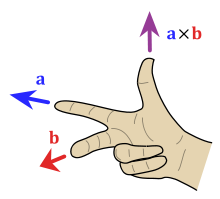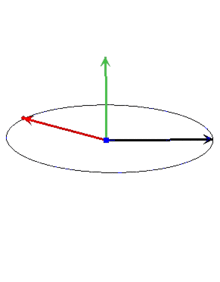另外还有一个有用的特征那就是叉积的绝对值就是A和B为两边说形成的平行四边形的面积。也就是AB所包围三角形面积的两倍。在计算面积时，我们要经常用到叉积。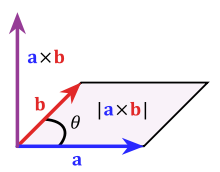（译注：三维及以上的叉乘参看维基：http://en.wikipedia.org/wiki/Cross_product）

点-线距离
找出一个点和一条线间的距离是经常遇见的几何问题之一。假设给出三个点，A，B和C，你想找出点C到点A、B定出的直线间距离。第一步是找出A到B的向量AB和A到C的向量AC，现在我们用该两向量的叉积除以|AB|，这就是我们要找的的距离了（下图中的红线）。
d = (AB x AC)/|AB|

如果你有基础的高中几何知识，你就知道原因了。上一节我们知道(AB X AC)/2是三角形ABC的面积，这个三角形的底是|AB|，高就是C到AB的距离。有时叉积得到的是一个负值，这种情况下距离就是上述结果的绝对值。
当我们要找点到线段的距离时，情况变得稍稍复杂一些。这时线段与点的最短距离可能是点到线段的某一端点，而不是点到直线的垂线。例如上图中点C到线段AB的最短距离应该是线段BC。我们有集中不同的方法来判断这种特殊情况。第一种情况是计算点积AB B来判定两线段间夹角。如果点积大于等于零，那么表示AB到BC是在-90到90度间，也就是说C到AB的垂线在AB外，那么AB上到C距离最近的点就是B。同样，如果BAAC大于等于零，那么点A就是距离C最近的点。如果两者均小于零，那么距离最近的点就在线段AB中的莫一点。

源代码参考如下：
//Compute the dot product AB   BC
int dot(int[] A, int[] B, int[] C){
AB = new int;
BC = new int;
AB = B-A;
AB = B-A;
BC = C-B;
BC = C-B;
int dot = AB * BC + AB * BC;
return dot;
}
//Compute the cross product AB x AC
int cross(int[] A, int[] B, int[] C){
AB = new int;
AC = new int;
AB = B-A;
AB = B-A;
AC = C-A;
AC = C-A;
int cross = AB * AC - AB * AC;
return cross;
}
//Compute the distance from A to B
double distance(int[] A, int[] B){
int d1 = A - B;
int d2 = A - B;
return sqrt(d1*d1+d2*d2);
}
//Compute the distance from AB to C
//if isSegment is true, AB is a segment, not a line.
double linePointDist(int[] A, int[] B, int[] C, boolean isSegment){
double dist = cross(A,B,C) / distance(A,B);
if(isSegment){
int dot1 = dot(A,B,C);
if(dot1 > 0)return distance(B,C);
int dot2 = dot(B,A,C);
if(dot2 > 0)return distance(A,C);
}
return abs(dist);
}

上面的代码看起来似乎是很繁琐。不过我们可以看看在C++和C#中，采用了运算符重载的类point，用‘*’代表点乘，用'^'代表叉乘（当然'+''-'还是你所希望的），那么看起来就简单些，代码如下：

//Compute the distance from AB to C
//if isSegment is true, AB is a segment, not a line.
double linePointDist(point A, point B, point C, bool isSegment){
double dist = ((B-A)^(C-A)) / sqrt((B-A)*(B-A));
if(isSegment){
int dot1 = (C-B)*(B-A);
if(dot1 > 0)return sqrt((B-C)*(B-C));
int dot2 = (C-A)*(A-B);
if(dot2 > 0)return sqrt((A-C)*(A-C));
}
return abs(dist);
}

展开全文pangshaohua 2019-02-25 14:06:01
• 1.向量点乘公式推导和几何解释 01.向量点乘(dot product)是其各个分量乘积的和，公式： 用连加号写： 02.几何解释： 点乘的结果是一个标量，等于向量大小与夹角的cos值的乘积。 a•b = |a||b|cosθ 如果a...

1.向量点乘公式推导和几何解释

01.向量点乘(dot product)是其各个分量乘积的和，公式：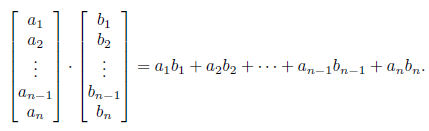用连加号写：

向量a=(a1*e1, a2*e2,a3*e3........an*en)其中e1，e2，e3.......en为正交规范基（俩俩正交，每个向量单位为1）

向量b=(b1*e1, b2*e2,b3*e3........bn*en)其中e1，e2，e3.......en为正交规范基（俩俩正交，每个向量单位为1）

则向量a.b有下面的公式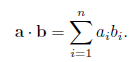02.几何解释：

点乘的结果是一个标量，等于向量大小与夹角的cos值的乘积。

a•b = |a||b|cosθ

如果a和b都是单位向量，那么点乘的结果就是其夹角的cos值。

a•b = cosθ

03.推导过程：

假设a和b都是二维向量，θ1是a与x轴的夹角，θ2是b与x轴的夹角，向量a与b的夹角θ等于θ1 - θ2.

a•b = ax*bx + ay*by

=  (|a|*sinθ1) * (|b| * sinθ2) +   (|a| * cosθ1) * (|b| * cosθ2)

= |a||b|(sinθ1*sinθ2 + cosθ1*cosθ2)

=|a||b|(cos(θ1-θ2))

= |a||b|cosθ

2.点乘交换率和分配率的推导

01.交换率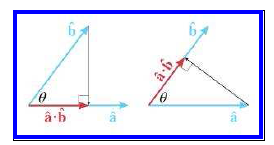02.分配率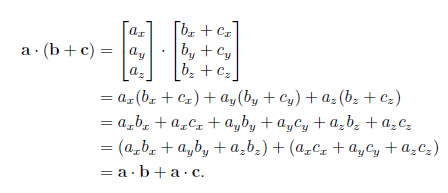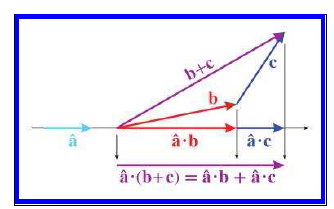注：更多内容参见：<3D math primer for graphics and game development  second edition>点击打开链接

展开全文qq_43193797 2020-03-19 00:12:59
• 向量点乘叉乘、矩阵、OpenGL变化 向量叉乘矩阵表示

向量点乘叉乘：点乘又叫向量的内积，叉乘又叫向量的外积。点乘计算得到的结果是一个标量；叉乘得到的结果是一个垂直于原向量构成平面的向量点乘：只能存在于2个向量之间的相乘。2个三维单元...

向量：向量就是在3D笛卡尔坐标中的一个顶点。单位向量就是长度为1的向量、

标量：标量是一个只有数值大小 没有方向，部分有征服之分。通俗来说标量只有大小没有方向的量。

向量和标量的区别就是 向量是有方向的。标量是没有方向的。

向量的点乘叉乘：点乘又叫向量的内积，叉乘又叫向量的外积。点乘计算得到的结果是一个标量；叉乘得到的结果是一个垂直于原向量构成平面的向量。

点乘：只能存在于2个向量之间的相乘。2个三维单元向量 之间进行点乘得到的是一个标量。 这个标量就表示为这两个向量之间的夹角。在我们开发中我们不需要进行向量之间的相乘。我们只需要去调用math3D库里面提供的点乘的API就可以了。方法有 1.float m3ddDotProduct3(const M3DVector3f u, const M3DVector3f v),这个方法我们得到的是两个向量点乘的结果。如果我们需要获取两个向量之间的角度。还需要对获得的数据进行转化。当然 API还提供了另外一个方法 可以直接获取到两个向量夹角的弧度方法2. float m3dGetAngleBetweenVector3(const M3DVector3f u , const M3DVector3d v),用这个方法我们可以直接获取到两个向量夹角的弧度。

叉乘：向量的叉乘获得到是另外一个新的向量。而这个向量是垂直于原来2个向量定义的平面垂直。叉乘和点乘不同。可以使用普通的向量相乘。如图所示当然 叉乘API也提供了相应的方法 void m3dCrossProduct3(M3DVector3f result,const M3DVector3f u,const M3DVector3f v);

矩阵：在空间中有一个点。使用xyz来描述它。如果我们要对这个点进行移动的，我们要知道这个点新的位置。那么我们就需要通过矩阵计算来获取新的位置。矩阵不仅仅3*3或者是4*4 的这种，如果这个矩阵 只有一行或者只有一列 也可以成为矩阵。我们也可以叫他为向量。

矩阵的叉乘：矩阵的叉乘是有一个规则。这个规则定义了什么样的2个矩阵可以进行叉乘。什么样2个矩阵不能进行叉乘。如图所示：下面的图片中有框框标记的数字如果相等那么这个两个矩阵就可以相乘。如果不相等那么这两个矩阵就不能相乘。在我们OPenGL的角度里。因为在OPenGL的约定里更多的是倾向使用一维数组。这样的原因是：OPenGL使用的是Column-Major(以列为主)矩阵排序的约定,所以在我们获取变化顶点向量的时候 使用的是矩阵左乘。

OPenGL的几种变换：

1.视图变换，即指定观察者的位置。视图变换是我们再应用场景中使用的第一种变化。简单来说 就是我们再观察一个物体的时候首先要确定观察的角度和观察的位置。只有先定义了观察者的位置 我们再对物体进行移动的时候才能观察到。

2.模型变换，就是用来操作物体通过某一种规律将物体移动到某一个位置，来进行旋转 缩放 平移，

3. 投影变换：分为正投影和透视投影。我们可以通过下面的图来感受一下这两种投影的区别：展开全文weixin_35857807 2020-12-31 11:23:54
• 向量点乘和叉乘 线性代数 Math

qq_16184125 2021-05-29 20:46:30
• wanguangtong 2019-11-26 10:54:06
• 向量点乘的含义 向量点乘

qq_28481231 2017-09-06 09:27:55
• Warmth_Dream 2020-09-09 19:48:11
• w_weixiaotao 2020-06-12 11:55:29
• weixin_45553106 2019-11-11 20:51:20
• 向量点乘相关公式推导 线性代数 向量

hb_zhouyj 2018-06-29 16:07:21
• qq_38913715 2021-04-27 18:23:08
• 向量点乘 three.js webgl

qq_39503511 2021-01-26 15:51:12
• Unity 通过向量点乘叉乘判断方位 unity 点乘叉乘

qq_42139931 2021-10-10 16:12:47
• qfikh 2019-11-16 20:00:30
• 向量点乘（内积）和叉乘（外积） 向量

liuxiao723846 2019-03-09 14:13:47
• weixin_39729272 2020-12-20 17:54:44
• 三角函数Cos求值，向量点乘 三角函数 向量

qq_25013675 2018-09-02 12:33:38
• Hodors 2021-01-14 17:58:28
• 向量点乘与向量叉乘的几何意义 向量点积 向量叉乘

qq826364410 2019-02-01 17:50:04
• racerun 2021-06-28 01:40:16
• weixin_39614094 2020-12-20 09:05:25
• 向量点乘与差乘的区别，以及python下np.dot函数 dot函数

HHTNAN 2018-01-04 13:23:31
• 向量点乘(即内积)和叉乘(即外积、向量积)区别与意义分析 线性代数 机器学习

Lu_gl 2022-01-05 19:24:57
• 向量点乘\叉乘，矩阵向量乘法 numpy

m0_46204224 2020-03-29 21:23:28
• weixin_30363817 2019-10-02 22:52:52
• Python线性代数学习笔记——向量的点乘与几何意义，实现向量的点乘操作 python向量点乘

weixin_39946429 2020-12-04 17:20:49...